# Test: Electronic Devices - 2

## 10 Questions MCQ Test GATE ECE (Electronics) 2023 Mock Test Series | Test: Electronic Devices - 2

Description
Attempt Test: Electronic Devices - 2 | 10 questions in 30 minutes | Mock test for Electronics and Communication Engineering (ECE) preparation | Free important questions MCQ to study GATE ECE (Electronics) 2023 Mock Test Series for Electronics and Communication Engineering (ECE) Exam | Download free PDF with solutions
QUESTION: 1

### Which of the following quantities cannot be measured/determined using Hall Effect?

Solution:

If a semiconductor carrying current is placed in a transverse magnetic field B, an electric field E is induced in the direction perpendicular to both I and B. This phenomenon is known as the Hall Effect, it can be used to determine whether semi-conductor is n-or p-type and to find the carrier concentration and mobility.

Diffusion constant is found from electron mobility using Einstein relationship.

*Answer can only contain numeric values
QUESTION: 2

### The ratio of hole diffusion current to the electron diffusion current in an infinite p-n junction is 4. If conductivity on p-side is 1.4 S/cm and on n-side is 2.8 S/cm, then the ratio of diffusion length Ln and Lp (ie Ln/Lp) is _______.

Solution:

For diffusion current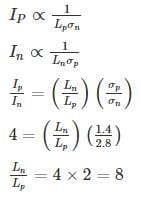QUESTION: 3

### Under high electric fields, in a semiconductor with increasing electric field,

Solution:

we known that

V = μE

μ = constant when E < 103 V/cm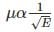when 103 < E < 104 V/cm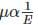when E > 104 V/cm

∴ When E Increase mobility decrease.

*Answer can only contain numeric values
QUESTION: 4

The energy band structure of n-type semiconductor is as shown below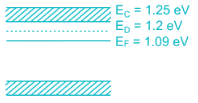The probability that the donor energy state is empty is (up to 3 significant digits)

Solution:

According to Fermi function,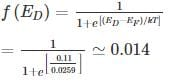Probability it is empty is 1 – F(ED) = 1 – 0.014 = 0.986

QUESTION: 5

In a hypothetical semiconductor hole concentration is 2 × 1015/cm3 and electron concentration is 1 × 1015/cm3. The mobility of holes is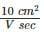and mobility of electrons is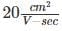If the specimen is subjected to hall measurement. Then the measured hall coefficient (RH) is

Solution:

For a semiconductor with significant concentration of both type of carriers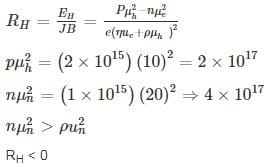*Answer can only contain numeric values
QUESTION: 6

In a germanium semiconductor at 127°C has a hole concentration gradient between two points 1.5 × 1022/m3. If the hole mobility in germanium is 800 cm2/v-s, then the diffusion current density is ______ A/m2.

Solution:

Given,

T = 127°C = 400°K

μP = 800 cm2/v-s

DP = μVT

VT = kT/2 =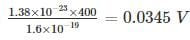⇒ DP = 800 × 0.0345 cm2/s = 27.6 cm2/s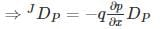= -1.5 × 1022 × 10-8 × 1.6 × 10-19 × 27.6 A/cm2

= -6.624 A/m2

QUESTION: 7

2 Consider two energy levels: E1, E eV above the Fermi level and E2, EeV below the Fermi level. P1 and P2 are respectively the probabilities of E1 being occupied by an electron and E2 being empty. Then

Solution:

Case 1:

E1 ⇒ EeV above the fermi level probability of E1 being occupied by on electron = P(E1)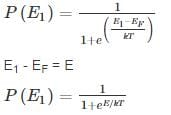Case II:

E2 → EeV below the fermi level probability of E2 being occupied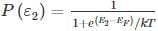Probability of E2 being empty: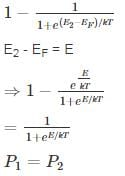*Answer can only contain numeric values
QUESTION: 8

A silicon sample is dope with unknown concentration of Arsenic, such that the fermi level (EF) is 0.407 eV relative to intrinsic level (Ei). The conductivity of the silicon sample after doping is______(Ω – cm)-1.

The mobility of electrons μn = 3800 cm2/v-s and holes μp = 1800 cm2/v-s. Take kT = 0.0259 eV at 300°K and intrinsic concentration ni = 1.5 × 1010 cm-3

Solution:

Given, Si sample is doped with As (donor impurity) and the band structure is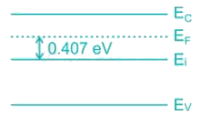Let nd be doping concentration, hence the electron concentration also is nd.

The extrinsic electron concentration is related to intrinsic concentration by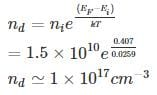∴ Conductivity σ = μndq

= 3800 × 1 × 1017 × 1.6 × 10-19

= 60.8 (Ω - cm)-1

QUESTION: 9

The effective density of states in conduction and valence band NC and Nv at 300K is 4.7x1017 cm−3 and 7x1018cm−3 respectively. The bandgap of the mentioned semiconductor is 1.42eV at 300K. The intrinsic carrier concentration is:

Use Boltzmann constant k = 8.62 x 10−5 eV/K

Solution:

Given
Nc = 4.7 x 1017 cm−3
Nv = 7 x 1018 cm−3

Eg = 1.42 eV
Now,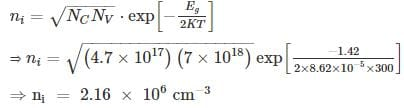*Answer can only contain numeric values
QUESTION: 10

Holes are being steadily injected into a region of n-type silicon. In the steady state, the excess hole concentration profile is shown. [The term excess means over and above concentrations pno]

If  Dp = 12 cm2/sec ND = 1016/cm3, ni = 1.5 × 1010/cm3 and w = 5 μm.

The current density that will flow in x-direction is _____ nA/cm2.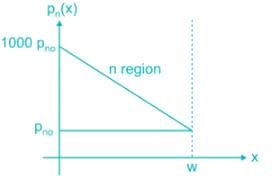Solution:

The diffusion current density is given by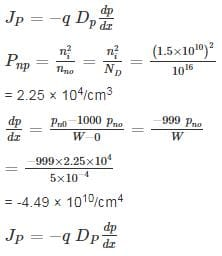= -4.49 × 1010/cm4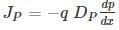= -1.6 × 10-19 × 12 × (-4.49 × 1010)

= 8.64 × 10-8 A/cm2

= 86.4 × 10-9 nA/cm2Use Code STAYHOME200 and get INR 200 additional OFF Use Coupon Code